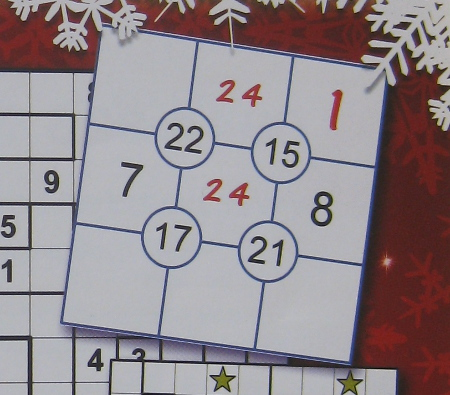# Sujiko Solver

This is a partially filled in Sujiko puzzle, taken from the cover of December’s Beyond Sudoku magazine.It’s a pretty straightforward puzzle - simply fill in the digits one to nine in the cells such that each set of four cells around the centre circles adds up to the number shown in that circle. There is more information on the inventor’s webpage here.

Since solving these puzzles is relatively easy, and having just finished reading this book</a>, I decided to try and come up with something more interesting to do with them. I had a number of questions that I wanted to answer…

Question - Is it possible to make a Sujiko puzzle that can still be solved without any clues being provided?

Answer - Yes, it is! In fact there are 1035 different ways (not counting symmetrical variations) that a no-clue puzzle can be made. For example, the puzzle with centre circle digits in clockwise order 10,14,22,19.

Question - How many different (again, not counting symmetrical variations) Sujiko puzzles exist?

Answer - Out of the 26,796 different possible combinations of the four circled central digits, 8607 of them give rise to a puzzle with one or more solutions.

Question - What is the largest number of solutions that a single puzzle (with no clues) can have?

Answer - There are two puzzle layouts that have 64 different solutions. This happens with either four 19’s in the centre circles or four 21’s.

NB. To turn a multiple solution puzzle into a single solution puzzle it is necessary to add some clues to narrow down the number of possible solutions.

So, where did I get all of these numbers from?

Firstly, you can use a bit of logic and some maths to work out how many different possible combinations of centre circled digits might make a puzzle. The centre circle digits can be a minimum of 10 (= 1 + 2 + 3 + 4) and a maximum of 30 ( = 6 + 7 + 8 + 9). This means a centre circle can be one of 21 different values. There is then some nice mathematics at this page showing the use of Burnside’s Lemma to count the number of different colourings of the corners of a triangle using a given number of colours. In our case we can use the result they derived for a square and set n=21, giving us the answer 26,796.

Since this isn’t an especially big number I decided it would be easy to write a computer program to exhaustively investigate all of these possibilities. I’d already realised that there are only 362,880 (= 9!) possible ways to fill in the digits one to nine on the grid. That’s nine ways to choose the first digit, multiplied by eight ways to choose the next digit from the remaining digits, multiplied by seven ways to choose the next digit … and so on.

The end result is a fairly simple bit of C++ code. It first tries out every possible variation of circled clues from 10 to 30 to make a set of all of the possible puzzles. I used a set so that I only stored one of each possible version of the puzzle. When checking to see if I’ve already seen this puzzle I try out all possible transformations (reflection, rotation, etc) on the puzzle first. There are 194,481 (= 21 ^ 4) different combinations and eight different symmetry transformations possible for each, which doesn’t take a modern computer long to check. At the end of this process I’m left with a list of all possible puzzle variants and it’s reassuring to see that the computer agrees with the mathematics, giving 26,796 different puzzles.

The slowest part of the process, taking around a minute and a half is to then try out each of the 362,880 possible solutions for each of the 26,796 puzzles and count up how many solutions each puzzle has, which ones only have a single solution, which has the most solutions, how many can/can’t be solved at all and so on. I’ve configured the code to print out each solvable puzzle, with a count of how many solutions it has.

In writing the “info dump” part of the program I’d already effectively written a Sujiko Solver, so I added an option to allow the program to be used as a solver. Instructions are included in the solver. The program has a few rough edges (eg checking the given clues against the possible solutions doubles the time needed for the info dumping. It’s an easy fix, but I didn’t bother to do it!) but seems to work OK.

If you are interested you can download a copy of the source code here.

Categories:

Updated: